Q.1) Fill in the missing place :
6 x 9 = ?
a) 48
b) 54
c) 60

Q.2) Fill in the missing place :
10 x 8 = ?
a) 100
b) 90
c) 80

Q.3) 13 x 3 = ?
a) 25
b) 39
c) 19

Q.4) 24 x 2 = ?
a) 54
b) 60
c) 48

Q.5) 46 x 2 = ?
a) 92
b) 100
c) 86

Q.6) 222 x 2 = ?
a) 430
b) 444
c) 462

Q.7) 366 x 2 = ?
a) 732
b) 756
c) 750

Q.8) 5 x 40 = ?
a) 100
b) 20
c) 200

Q.9) 5 x 300 = ?
a) 1500
b) 150
c) 750

Q.10) 22 x 14 = ?
a) 294
b) 308
c) 326

### Multiplication Worksheets Grade 3 Explanations

Q.1) Explanation – Multiplication Worksheets Grade 3

According to the Table of 6If we look at the Table, we would get 6 x 9 = 54

Q.2) Explanation – Multiplication Worksheets Grade 3

According to the Table of 10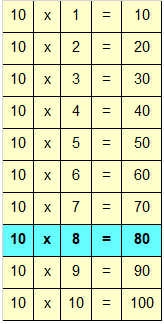If we look at the Table, we would get 10 x 8 = 80

Q.3) Explanation – Multiplication Worksheets Grade 3

Step I: Multiply 3 in ones place by 3
3 x 3 = 9
Write 9 in ones place.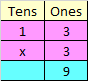Step II: Multiply 1 in tens place by 3
1 x 3 = 3
Write 3 in tens place.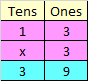Hence, 13 x 3 = 39

Q.4) Explanation – Multiplication Worksheets Grade 3

Step I: Multiply 4 in ones place by 2
4 x 2 = 8
Write 8 in ones place.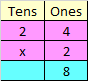Step II: Multiply 2 in tens place by 2
2 x 2 = 4
Write 4 in tens place.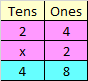Hence, 24 x 2 = 48

Q.5) Explanation – Multiplication Worksheets Grade 3

Step I: Multiply 6 in ones place by 2
6 x 2 = 12
Since, the number is more than 9, we need to carry over 1 to the tens place.
Write 2 at ones place.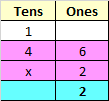Step II: Multiply 4 in tens place by 2
4 x 2 = 8
Add the carry over of 1 to 8
8 + 1 = 9
Write 9 in tens place.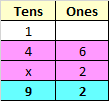Hence, 46 x 2 = 92

Q.6) Explanation – Multiplication Worksheets Grade 3

Step I: Multiply 2 in ones place by 2
2 x 2 = 4
Write 4 in ones place.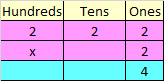Step II: Multiply 2 in tens place by 2
2 x 2 = 4
Write 4 in tens place.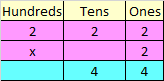Step III: Multiply 2 in hundreds place by 2
2 x 2 = 4
Write 4 in hundreds place.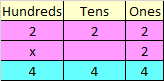Hence, 222 x 2 = 444

Q.7) Explanation – Multiplication Worksheets Grade 3

Step I: Multiply 6 in ones place by 2
6 x 2 = 12
Since, the number is more than 9, we need to carry over 1 to the tens place
Write 2 in ones place.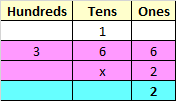Step II: Multiply 6 in tens place by 2
6 x 2 = 12
Add the carry over of 1 to 12
12 + 1 = 13
Since, the number is more than 9, we need to carry over 1 to the hundreds place
Write 3 in tens place.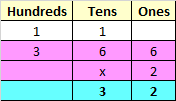Step III: Multiply 3 in hundreds place by 2
3 x 2 = 6
Add the carry over of 1 to 6
6 + 1 = 7
Write 7 in hundreds place.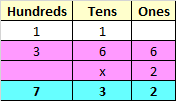Hence, 366 x 2 = 732

Q.8) Explanation – Multiplication Worksheets Grade 3

To multiply Multiplicand, with Multiplier having zero at the end, we first count the number of zeroes

In 40 there is 1 zero
Now we would multiply, the remaining digits of the given numbers
i.e
Multiplying 5 by 4
5 x 4 = 20
Add 1 zero after the product 20
It would become 200
Hence
5 x 40 = 200

Q.9) Explanation – Multiplication Worksheets Grade 3

To multiply Multiplicand, with Multiplier having zero at the end, we first count the number of zeroes

In 300 there are 2 zeros
Now we would multiply, the remaining digits of the given numbers
i.e
Multiplying 5 by 3
5 x 3 = 15
Add 2 zeros after the product 15
It would become 1500
Hence
5 x 300 = 1500

Q.10) Explanation – Multiplication Worksheets Grade 3

Step I: Whenever we multiply a two digit number by another 2 digit number, we first multiply the first number by ones place of second number, and then by first number by Tens place of the second number.
First we need to multiply 22 with 4
Multiply 4 by 2 in ones place.
4 x 2 = 8
Write 8 in ones place.
Multiply 4 by 2 in tens place.
4 x 2 = 8
Write 8 in tens place.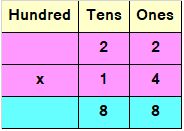Step II: Since the second number is a Tens value, we add a 0 or a cross to the answer obtained by multiplying first number by second digit

Multiply 22 with 1 and before multiplying write ‘x’ under 8

Multiply 1 by 2 in ones place.

1 x 2 = 2

Write 2 in tens place under 8

Multiply 1 by 2 in tens place.

1 x 2 = 2

Write 2 in hundreds place before 2Step III: Now add both the parts which have been multiplied.

8 + 0 = 8

8 + 2 = 10

Since, the number is more than 9, we need to carry over 1 to the hundreds place.

Add hundreds place and the carry of 1

2 + 1 = 3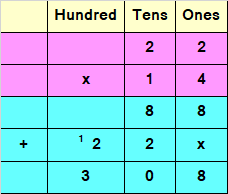Hence, 22 x 14 = 308